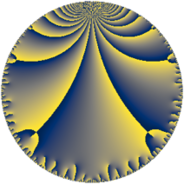Properties

 Label 19.7.fLevel $19$ Weight $7$ Character orbit 19.f Rep. character $\chi_{19}(2,\cdot)$ Character field $\Q(\zeta_{18})$ Dimension $54$ Newform subspaces $1$ Sturm bound $11$ Trace bound $0$

Related objects

Defining parameters

 Level: $$N$$ $$=$$ $$19$$ Weight: $$k$$ $$=$$ $$7$$ Character orbit: $$[\chi]$$ $$=$$ 19.f (of order $$18$$ and degree $$6$$) Character conductor: $$\operatorname{cond}(\chi)$$ $$=$$ $$19$$ Character field: $$\Q(\zeta_{18})$$ Newform subspaces: $$1$$ Sturm bound: $$11$$ Trace bound: $$0$$

Dimensions

The following table gives the dimensions of various subspaces of $$M_{7}(19, [\chi])$$.

Total New Old
Modular forms 66 66 0
Cusp forms 54 54 0
Eisenstein series 12 12 0

Trace form

 $$54 q - 6 q^{2} - 36 q^{3} + 192 q^{4} - 6 q^{5} + 720 q^{6} - 219 q^{7} - 9 q^{8} - 2076 q^{9} + O(q^{10})$$ $$54 q - 6 q^{2} - 36 q^{3} + 192 q^{4} - 6 q^{5} + 720 q^{6} - 219 q^{7} - 9 q^{8} - 2076 q^{9} - 1689 q^{10} + 1677 q^{11} - 9 q^{12} - 10506 q^{13} + 6105 q^{14} + 24138 q^{15} + 23760 q^{16} - 3438 q^{17} - 30918 q^{19} - 76650 q^{20} - 59055 q^{21} + 20490 q^{22} + 42498 q^{23} + 31848 q^{24} + 7914 q^{25} + 109989 q^{26} + 124821 q^{27} - 109476 q^{28} - 27726 q^{29} - 153516 q^{30} - 30789 q^{31} - 114375 q^{32} + 126039 q^{33} + 359796 q^{34} + 160161 q^{35} + 184995 q^{36} - 245244 q^{38} - 390564 q^{39} - 372678 q^{40} - 24708 q^{41} - 244893 q^{42} - 30726 q^{43} - 301419 q^{44} + 293037 q^{45} + 69246 q^{46} - 71454 q^{47} + 1024041 q^{48} - 350346 q^{49} - 104148 q^{50} - 180672 q^{51} + 921801 q^{52} + 822558 q^{53} + 308469 q^{54} + 623517 q^{55} - 17862 q^{57} - 1150644 q^{58} - 463812 q^{59} - 908130 q^{60} - 583698 q^{61} - 429732 q^{62} - 2576043 q^{63} - 328293 q^{64} - 1056789 q^{65} - 1038756 q^{66} + 1120464 q^{67} + 717270 q^{68} + 3771567 q^{69} + 4845345 q^{70} + 94494 q^{71} + 2351634 q^{72} + 950850 q^{73} + 55149 q^{74} - 218814 q^{76} - 343086 q^{77} + 1833471 q^{78} - 4936494 q^{79} - 9642039 q^{80} - 769551 q^{81} - 8627793 q^{82} - 412131 q^{83} - 3125205 q^{84} - 896910 q^{85} + 8878236 q^{86} + 610737 q^{87} + 13604751 q^{88} + 6653022 q^{89} + 3142734 q^{90} + 3409821 q^{91} - 3043740 q^{92} - 816393 q^{93} + 4839186 q^{95} - 5667138 q^{96} - 853656 q^{97} - 17679315 q^{98} - 13991937 q^{99} + O(q^{100})$$

Decomposition of $$S_{7}^{\mathrm{new}}(19, [\chi])$$ into newform subspaces

Label Dim. $$A$$ Field CM Traces $q$-expansion
$a_{2}$ $a_{3}$ $a_{5}$ $a_{7}$
19.7.f.a $54$ $4.371$ None $$-6$$ $$-36$$ $$-6$$ $$-219$$